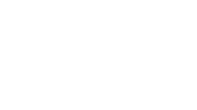# Solutions

Get detailed explanations to advanced GMAT questions.

### QuestionThe outside of the rectangular box represented in the figure above is to be decorated by attaching pieces of wrapping paper to cover all surfaces except the bottom of the box. What is the minimum number of square inches of wrapping paper needed?

Option A:

375

Option B:

600

Option C:

725

Option D:

800

Option E:

1250

Easy

### Solution

Option B is the correct answer.

### Option Analysis

Total area of cuboid – area of bottom of cuboid = paper required to wrap all sides except bottom of the cuboid

Total area of cuboid = area of top and bottom side faces (l*b)+area of two side faces(h*b) + area of two side faces (l*h) = 2*25*10 + 2*5*10 + 2*25*5 = 500+100+250=850

Area of bottom face = 250

So paper needed = 850 – 250 = 600 square inches.